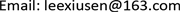﻿ 基于供应链服务水平优化的双边际化效应分析 Double Marginalization Analyzing Based on Service Level in Supply Chain

Service Science and Management
Vol. 07  No. 05 ( 2018 ), Article ID: 26992 , 5 pages
10.12677/SSEM.2018.75018

Double Marginalization Analyzing Based on Service Level in Supply Chain

Xiusen Li, Yuejun Pan, Xiaoyi Wang

School of Mathematics and Statistics, Shandong University of Technology, Zibo ShandongReceived: Sep. 6th, 2018; accepted: Sep. 21st, 2018; published: Sep. 28th, 2018ABSTRACT

Analysis of double marginalization based on service level optimizing of joint decision, is studied in supply chain with a vendor and a retailer. The conclusion is that join decision greatly effects double marginalization, and rational contract decision based on cost sharing weakens double marginalization on the chain’s profitability.

Keywords:Supply Chain, Cycle Service Level (CSL), Double Marginalization, Cost of SurplusCopyright © 2018 by authors and Hans Publishers Inc.1. 引言

2. 基于成本分担的周期服务水平优化

P——零售价；c——供货价；m——生产成本；r——单位销售剩余由零售商处理的价格( $0 )；b——单位销售剩余退货给供应商处理的价格( $0 )；h——单位产品一周期的库存成本(假设 $p-c-h>0$ )。

u——供小于求时零售商承担的单位缺货损失(Cost of under-stocking)，假设缺货意味顾客丢失，即 $u=p-c-h/2$，其中h/2为周期内已销售部分的平均库存成本。

v——供过于求时单位销售剩余成本(Cost of overstocking)，包括单位销售剩余产品(以下简称“剩余”)的库存成本和处理成本。合作模式如下：单位剩余的库存成本h在供销双方之间的分配因子为 $\beta$，零售商分担 $\beta h$，供应商分担 $\left(1-\beta \right)h$；单位剩余的处理成本的分配因子为 $\gamma$，零售商处理剩余时，零售商分担 $\gamma \left(c-r\right)$，此时零售商承担 $v=\beta h+\gamma \left(c-r\right)$；剩余退货给供应商处理时，零售商分担 $\gamma \left(m-b\right)$，此时零售商承担 $v=\beta h+\gamma \left(m-b\right)$$\left(0\le \beta ,\gamma \le 1\right)$

2.1. 零售商决策的最优服务水平

$CS{L}^{*}=u/\left(u+v\right)$ (1)

$CS{L}_{\text{R}}^{*}=\frac{u}{u+\beta h+\gamma \left(c-r\right)}$ (2)

$CS{L}_{\text{V}}^{*}=\frac{u}{u+\beta h+\gamma \left(m-b\right)}$ (3)

2.2. 整体决策的最优服务水平

$CS{L}_{\text{SCR}}^{*}=\frac{p-m-h/2}{p-r+h/2}$ (4)

$CS{L}_{\text{SCV}}^{*}=\frac{p-m-h/2}{p-b+h/2}$ (5)

2.3. 最优服务水平下的订货策略

${\pi }_{i}\left({Q}_{i}\right)={\int }_{{Q}_{i}}^{\infty }{Q}_{i}U{f}_{i}\left({x}_{i}\right)\text{d}{x}_{i}+{\int }_{0}^{{Q}_{i}}\left[{x}_{i}U-\left({Q}_{i}-{x}_{i}\right)V\right]{f}_{i}\left({x}_{i}\right)\text{d}{x}_{i}$

${Q}_{i}^{*}={F}_{i}^{-1}\left(CS{L}^{*},{\mu }_{i},{\sigma }_{i}\right)$ (6)

${F}_{i}^{-1}\left(\cdot \right)$${F}_{i}\left(\cdot \right)$ 反函数， $i=1,2,\cdots$ (证明略)。

3. 基于服务水平优化的风险成本分析

4. 基于服务水平优化的双边际化分析

4.1. 零售商处理剩余时双边际化分析

1) 不合作模式

$CS{L}_{\text{R}}^{\text{0}}=\frac{p-c-h/2}{p-r+h/2}$ (7)

2) 合作模式

3) 算例1

4.2. 供应商处理剩余时的边际化分析

1) 不合作模式和折价退货策略

$CS{L}_{\text{V}}^{\text{0}}=\frac{p-c-h/2}{p-c+h/2}$ (8)

$CS{L}_{\text{VD}}^{\text{0}}=\frac{p-c-h/2}{p-d+h/2}$ 。因为d < c，所以 $CS{L}_{\text{VD}}^{\text{0}}，即“折价退货策略”可以抑制零售商盲目提高服务水平，从而减弱这种盲目决策对于整体的伤害。

2) 合作模式

3) 算例2

5. 结束语

Double Marginalization Analyzing Based on Service Level in Supply Chain[J]. 服务科学和管理, 2018, 07(05): 151-155. https://doi.org/10.12677/SSEM.2018.75018

1. 1. Chopra, S. and Meindl, P. (2001) Supply Chain Management—Strategy, Planning, and Operation. Tsinghua University Publisher, Beijing, 183-229.

2. 2. 马云高, 王熊民. 消费者预测行为对供应链牛鞭效应和零售商库存的影响分析[J]. 运筹与管理, 2012, 21(5): 22-27.

3. 3. 申成霖, 张新鑫, 卿志琼. 服务水平约束下基于顾客策略性退货的供应链契约协调研究[J]. 中国管理科学, 2010, 18(4): 56-64.

4. 4. Wang, S.J., Huang, C.T., Wang, W.L., et al. (2010) Incorporating ARIMA Forecasting and Service-Level Based Replenishment in RFID-Enabled Supply Chain. International Journal of Production Research, 48, 2655-2677. https://doi.org/10.1080/00207540903564983

5. 5. 陈旭. 需求信息更新条件下易逝性品的订货批量策略[J]. 管理科学学报, 2003, 5(8): 38-42.

6. 6. 申成霖, 侯文华, 张新鑫, 卿志琼. 基于信息更新与服务水平约束的供应链订货及协调决策[J]. 中国管理科学, 2012, 20(5): 55-63.

7. 7. 吴育华, 赵强, 王初. 基于多人合作理论的供应链库存利益分配机制研究[J]. 中国管理科学, 2002, 12(10): 44-47.